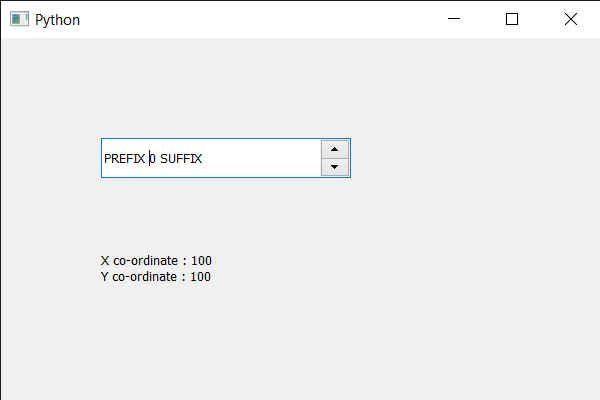# PyQt5 QSpinBox – How to get the X and Y co-ordinates respectively

• Last Updated : 28 May, 2020

In this article we will see how we can get the X and Y co-ordinates of the spin box. X co-ordinate is the distance of spin box from the left side of window frame and Y co-ordinate is the distance of spin box fro the upper side of the window frame, using X and Y co-ordinate we can determine the position of the spin box in the window.

In order to do this we use `x` and `y` method respectively with the spin box object.

Attention geek! Strengthen your foundations with the Python Programming Foundation Course and learn the basics.

To begin with, your interview preparations Enhance your Data Structures concepts with the Python DS Course. And to begin with your Machine Learning Journey, join the Machine Learning - Basic Level Course

Syntax : spin_box.x() and spin_box.y()

Argument : It takes no argument

Return : It returns integer

Below is the implementation

 `# importing libraries``from` `PyQt5.QtWidgets ``import` `*` `from` `PyQt5 ``import` `QtCore, QtGui``from` `PyQt5.QtGui ``import` `*` `from` `PyQt5.QtCore ``import` `*` `import` `sys`` ` ` ` `class` `Window(QMainWindow):`` ` `    ``def` `__init__(``self``):``        ``super``().__init__()`` ` `        ``# setting title``        ``self``.setWindowTitle(``"Python "``)`` ` `        ``# setting geometry``        ``self``.setGeometry(``100``, ``100``, ``600``, ``400``)`` ` `        ``# calling method``        ``self``.UiComponents()`` ` `        ``# showing all the widgets``        ``self``.show()`` ` `        ``# method for widgets``    ``def` `UiComponents(``self``):``        ``# creating spin box``        ``self``.spin ``=` `QSpinBox(``self``)`` ` `        ``# setting geometry to spin box``        ``self``.spin.setGeometry(``100``, ``100``, ``250``, ``40``)`` ` `        ``# setting range to the spin box``        ``self``.spin.setRange(``0``, ``9``)`` ` `        ``# setting prefix to spin``        ``self``.spin.setPrefix(``"PREFIX "``)`` ` `        ``# setting suffix to spin``        ``self``.spin.setSuffix(``" SUFFIX"``)`` ` `        ``# creating a label``        ``self``.label ``=` `QLabel(``self``)`` ` `        ``# making label multi line``        ``self``.label.setWordWrap(``True``)`` ` `        ``# setting label geometry``        ``self``.label.setGeometry(``100``, ``200``, ``120``, ``60``)`` ` `        ``# getting the X and Y co-ordinates``        ``x_value ``=` `self``.spin.x()``        ``y_value ``=` `self``.spin.y()`` ` `        ``# setting text to the label``        ``self``.label.setText(``"X co-ordinate : "` `+` `str``(x_value) ``+``                           ``" Y co-ordinate : "` `+` `str``(y_value))`` ` ` ` `# create pyqt5 app``App ``=` `QApplication(sys.argv)`` ` `# create the instance of our Window``window ``=` `Window()`` ` `# start the app``sys.exit(App.``exec``())`

Output :My Personal Notes arrow_drop_up